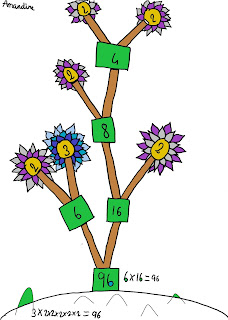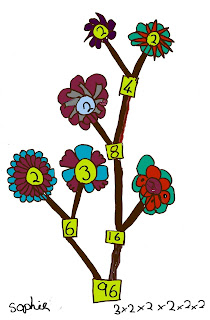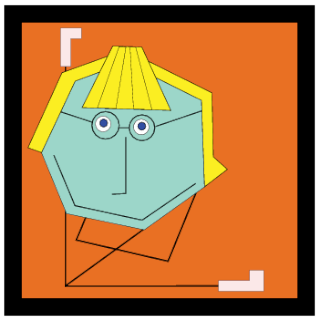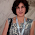## Monday, 4 February 2013

### Important factors

People normally draw factor trees like this:
This isn't right in three ways:
1. First of all, it should be a tree that goes up and actually looks like a tree.
2. As the prime factors are so important, they should stand out.
3. And  - the trees should be a bit more beautiful than this.
We started off in Year 5 looking at factors using the Cuisenaire rods, to make the factor walls of numbers. This makes what a factor is so clear:

Then we drew some different factor trees to investigate the question "What different factor trees can you make for 96?" -Next, we thought about what mathematical questions about prime numbers we could investigate. Here are some of them:

Ella-May - Do all numbers have a two and a three as one of their prime factors?

Mr Gregg - Are there any other numbers less than 100 with six prime factors?

Sophie - Would an odd number have as many prime factors as an even number?

William - Do bigger numbers have more prime factors?

Harry - Do bigger numbers have bigger prime factors?

Some children suggested we make a huge forest of trees for lots of numbers. Here's part of it:

You can see a bigger version here.

At the same time we played a game, Ocean of Primes, adapted from the nrich Factor Track:

(You can get this as a Word document here. Best to scale it up to A3 when you print it.)

To round off this term's work on factorisation we looked at a great book, Richard Evan Schwartz's You Can Count on Monsters

In this book, all the prime numbers are monsters with particular shapes:TwoThreeFiveSeven
Other numbers have designs made up of these ones. For instance, here's 14:14 has 7 and 2 as prime factors, so they're in the picture
We had a good look at the book and the poster:

After we'd absorbed what was going on, we created some of our own in a similar style:
Each has the prime factors of the number illustrated:Anna: 24 has the prime factors 3, 2, 2 and 2Emily: 104 has 13, 2, 2 and 2 as prime factorsSophie: The prime factors of 144 are 3, 2, 2, 3, 2, 2
UPDATE - FEBRUARY 2016 - THOUGHT FLOWERS

1.Mr. Gregg,

2.3.